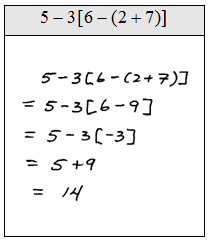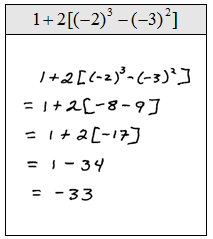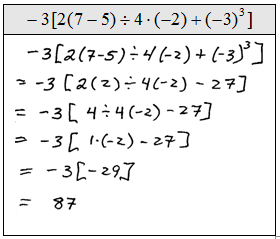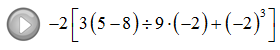## Algebra

Showing posts with label multiply. Show all posts
Showing posts with label multiply. Show all posts

### Multiplying Polynomials

The distributive property and the product rule for exponents are the keys to multiplication of polynomials.

Remember the product rule,It says that when we multiply two numbers with the same base we must add the exponents.

Monomial x PolynomialMultiply.A common mistake is to distribute when working with multiplication. The distributive property only applies when addition or subtraction separates the terms.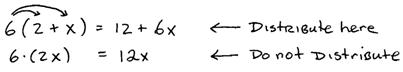Binomial x Polynomial: Use the distributive property to multiply polynomials by binomials.We can save a step by distributing each term in the binomial individually. Sometimes this technique is referred to as the FOIL method. (FOIL – Multiply the First, Outer, Inner and then Last terms together.)

Multiply.When combining like terms, be sure that the variable parts are exactly the same. Go slow and work in an organized fashion because it is easy to make an error when many terms are involved.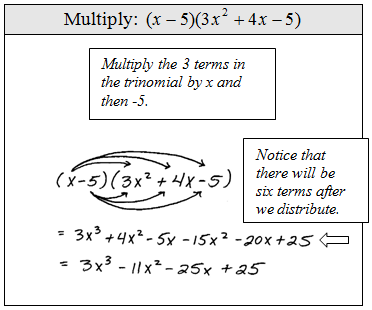It is good practice to recheck your distributive step.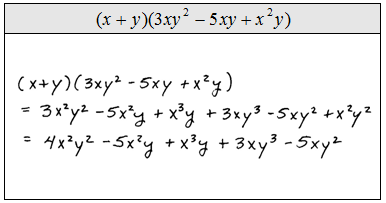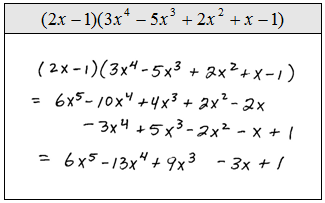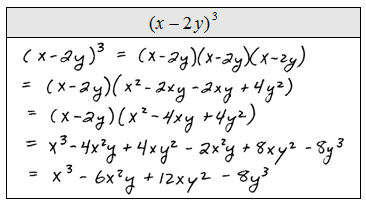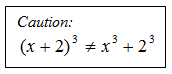Trinomial x Polynomial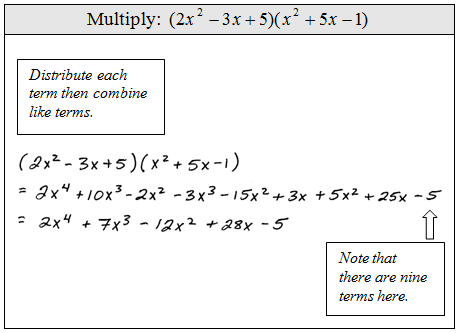The following problem is one that we will have to be able to do before moving on to the next algebra course. These are tedious, time consuming, and often worked incorrectly. Use caution, take your time and work slowly.Function notation for multiplication looks likeGiven the functions f and g find (f g)(x).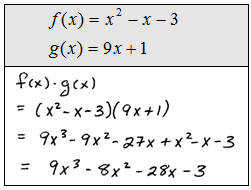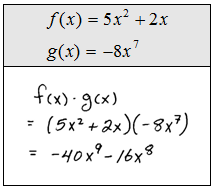The following special products will simplify things if we memorize them.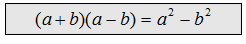This special product is often called difference of squares. Notice that the middle terms cancel because one term will always be positive and the other will be negative.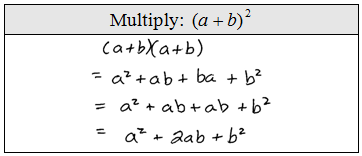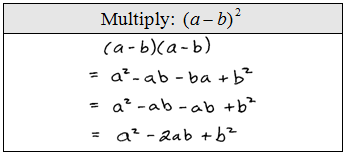We may use these formulas as templates when multiplying binomials. It is a good idea to memorize them.

Multiply.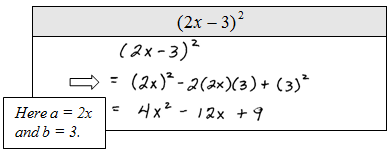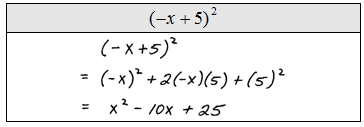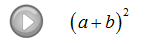### Order of Operations

When several operations are to be applied within a calculation, we must follow a specific order to ensure a single correct result.

Order of Operations:
1. Perform all calculations within the innermost Parentheses first.
2. Evaluate Exponent expressions.
3. Apply the Multiplication and Division operations from left to right.
4. Lastly, work all Addition and Subtraction operations from left to right.
Caution:  Please do not dismiss the fact that multiplication and division should be worked from left to right.  Many standardized exams will test you on this fact.  The following example illustrates the problem.Order of Operations - The Basics

Simplify.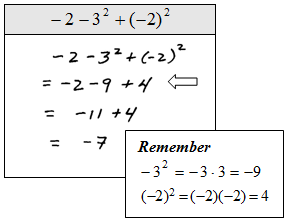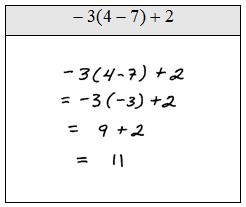Order of operation problems get a bit more tedious when fractions are involved. Remember that when adding or subtracting fractions you need to first find the equivalent fractions with a common denominator. Multiplication does not require a common denominator.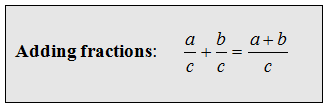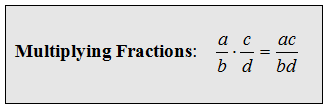Simplify.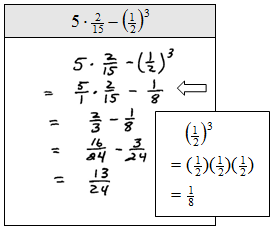The Order of Operations

We will see that some of the problems have different looking parentheses { [ ( ) ] }, treat them the same and just remember to perform the innermost parentheses first.  Some problems may involve an absolute value, in which case you will need to apply the innermost absolute value first as you would if it were a parentheses.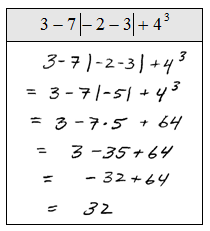To avoid these unnecessary mistakes, work one operation at a time and for each step rewrite everything.  This may seem like a lot of work but it really helps avoid errors.

Simplify.